Question

# The Evanec Company's next expected dividend, D1, is \$3.77; its growth rate is 6%; and its...

The Evanec Company's next expected dividend, D1, is \$3.77; its growth rate is 6%; and its common stock now sells for \$31.00. New stock (external equity) can be sold to net \$24.80 per share.

1. What is Evanec's cost of retained earnings, rs? Do not round intermediate calculations. Round your answer to two decimal places.

rs =   %

2. What is Evanec's percentage flotation cost, F? Round your answer to two decimal places.

F =   %

3. What is Evanec's cost of new common stock, re? Do not round intermediate calculations. Round your answer to two decimal places.

re =   %

a) calculation of cost of retained earnings:

Cost of retained earnings= (D1/current price)+ growth

Cost of retained earnings= (3.77/31)+0.06= 0.1216+0.06= 0.1816

Cost of retained earnings= 18.16%

b) calculation of flotation cost percentage:

Floatation cost percentage= (31-24.80)/31*100= 20%

c) calculation of cost of new stock:

Cost of new stock= (3.77/24.80)+0.06= 0.1520+0.06= 0.2120

Cost of new stock is 21.20%

#### Earn Coins

Coins can be redeemed for fabulous gifts.

Similar Homework Help Questions
• ### The Evanec Company's next expected dividend, D1, is \$3.10; its growth rate is 6%; and its...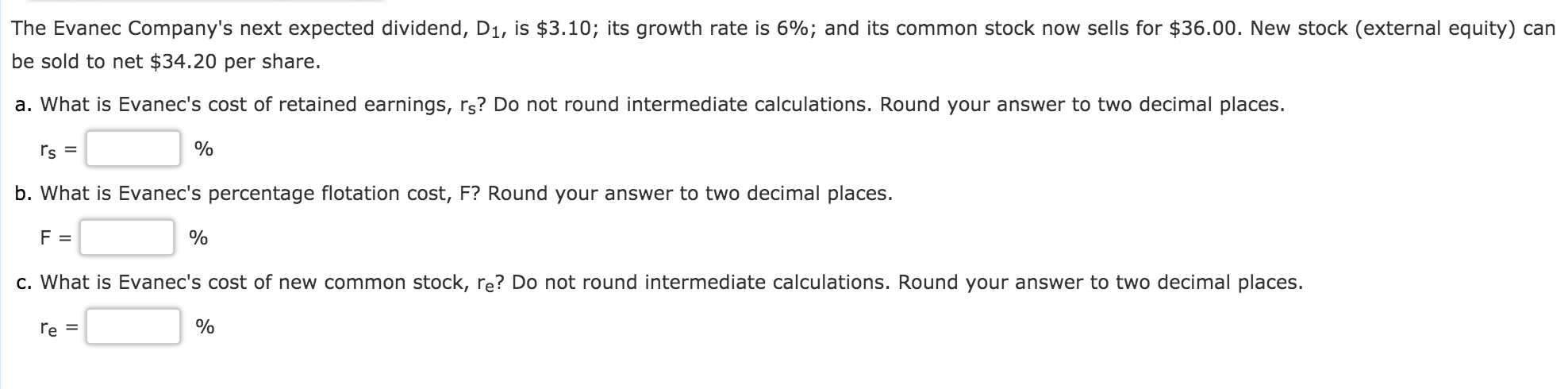The Evanec Company's next expected dividend, D1, is \$3.10; its growth rate is 6%; and its common stock now sells for \$36.00. New stock (external equity) can be sold to net \$34.20 per share. a. What is Evanec's cost of retained earnings, rs? Do not round intermediate calculations. Round your answer to two decimal places. rs = % | b. What is Evanec's percentage flotation cost, F? Round your answer to two decimal places. F = % c. What is...

• ### 6. The Evanec Company's next expected dividend, D1, is \$2.62; its growth rate is 7%; and...

6. The Evanec Company's next expected dividend, D1, is \$2.62; its growth rate is 7%; and its common stock now sells for \$37.00. New stock (external equity) can be sold to net \$33.30 per share. What is Evanec's cost of retained earnings, rs? Do not round intermediate calculations. Round your answer to two decimal places. rs =   % What is Evanec's percentage flotation cost, F? Round your answer to two decimal places. F =   % What is Evanec's cost of new common...

• ### eBook The Evanec Company's next expected dividend, D1, is \$3.06; its growth rate is 6%; and...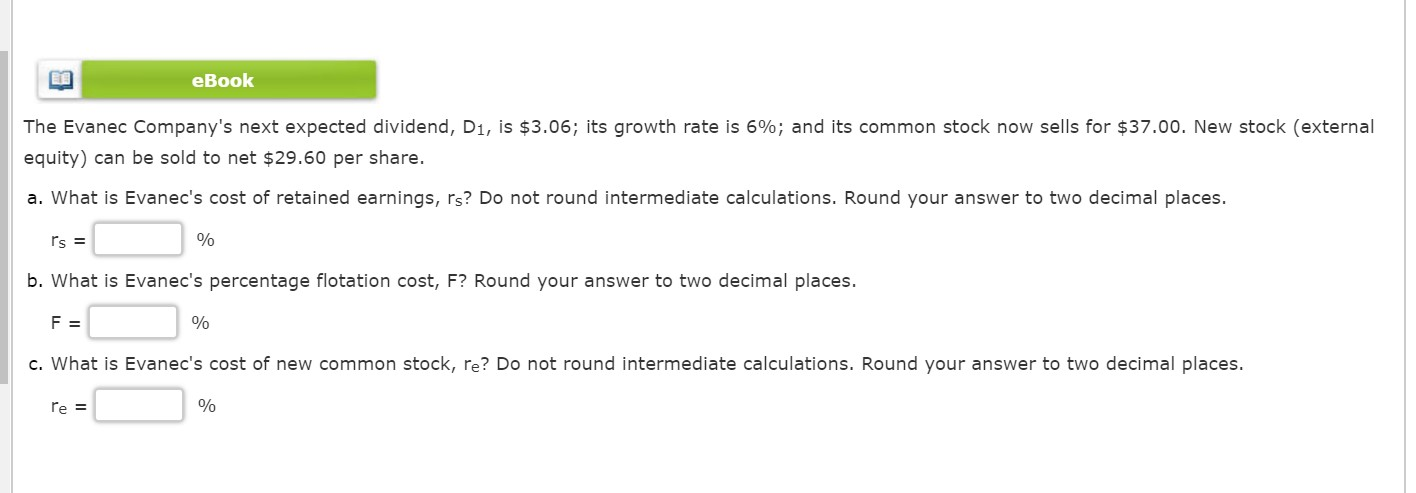eBook The Evanec Company's next expected dividend, D1, is \$3.06; its growth rate is 6%; and its common stock now sells for \$37.00. New stock (external equity) can be sold to net \$29.60 per share. a. What is Evanec's cost of retained earnings, rs? Do not round intermediate calculations. Round your answer to two decimal places. rs = b. What is Evanec's percentage flotation cost, F? Round your answer to two decimal places. F = 이이 c. What is Evanec's...

• ### The Evanec Company's next expected dividend, D1, is \$2.92; its growth rate is 4%; and its...

The Evanec Company's next expected dividend, D1, is \$2.92; its growth rate is 4%; and its common stock now sells for \$37.00. New stock (external equity) can be sold to net \$33.30 per share. What is Evanec's cost of retained earnings, rs? Do not round intermediate calculations. Round your answer to two decimal places. rs =   % What is Evanec's percentage flotation cost, F? Round your answer to two decimal places. F =   % What is Evanec's cost of new common stock,...

• ### The Evanec Company's next expected dividend, D1, is \$3.44; its growth rate is 5%; and its...

The Evanec Company's next expected dividend, D1, is \$3.44; its growth rate is 5%; and its common stock now sells for \$30.00. New stock (external equity) can be sold to net \$27.00 per share. What is Evanec's cost of retained earnings, rs? Do not round intermediate calculations. Round your answer to two decimal places. rs =   % What is Evanec's percentage flotation cost, F? Round your answer to two decimal places. F =   % What is Evanec's cost of new common stock,...

• ### The Evanec Company's next expected dividend, D1, is \$2.91; its growth rate is 6%; and its...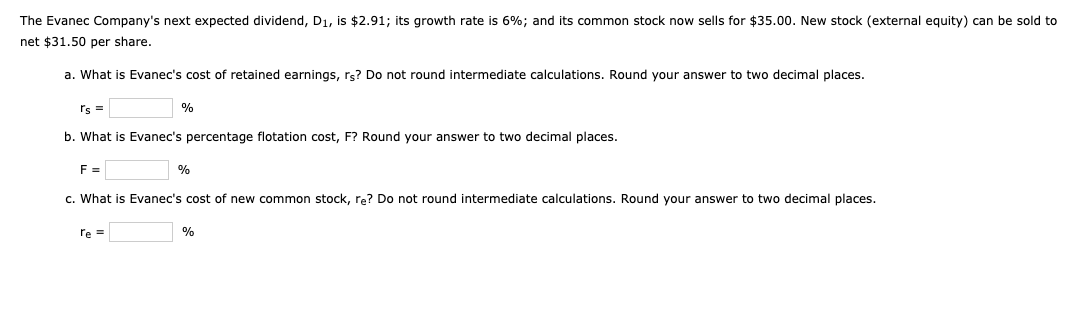The Evanec Company's next expected dividend, D1, is \$2.91; its growth rate is 6%; and its common stock now sells for \$35.00. New stock (external equity) can be sold to net \$31.50 per share. a. What is Evanec's cost of retained earnings, rs? Do not round intermediate calculations. Round your answer to two decimal places. Is = % b. What is Evanec's percentage flotation cost, F? Round your answer to two decimal places. F= % c. What is Evanec's cost...

• ### The Evanec Company's next expected dividend, D1, is \$2.76; its growth rate is 4%; and its...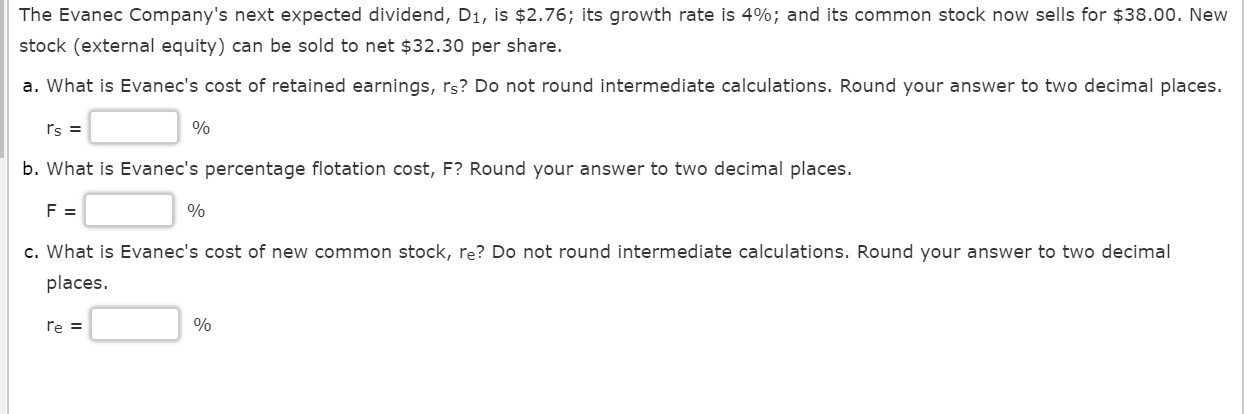The Evanec Company's next expected dividend, D1, is \$2.76; its growth rate is 4%; and its common stock now sells for \$38.00. New stock (external equity) can be sold to net \$32.30 per share. a. What is Evanec's cost of retained earnings, rs? Do not round intermediate calculations. Round your answer to two decimal places. Is = % b. What is Evanec's percentage flotation cost, F? Round your answer to two decimal places. F = % c. What is Evanec's...

• ### The Evanec Company's next expected dividend, D1, is \$3.90; its growth rate is 4%; and its...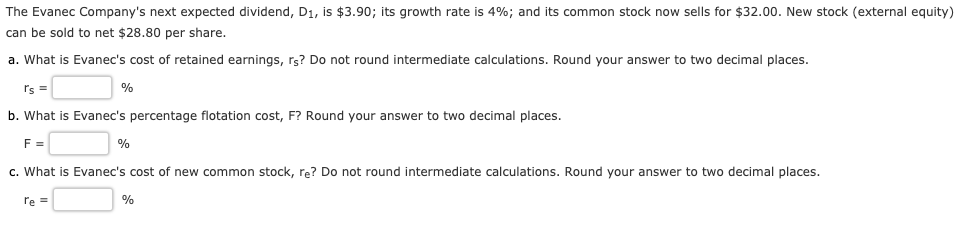The Evanec Company's next expected dividend, D1, is \$3.90; its growth rate is 4%; and its common stock now sells for \$32.00. New stock (external equity) can be sold to net \$28.80 per share. a. What is Evanec's cost of retained earnings, rs? Do not round intermediate calculations. Round your answer to two decimal places. r's = % b. What is Evanec's percentage flotation cost, F? Round your answer to two decimal places. F= % c. What is Evanec's cost...

• ### The Evanec Company's next expected dividend, D1, is \$2.86; its growth rate is 7%; and its...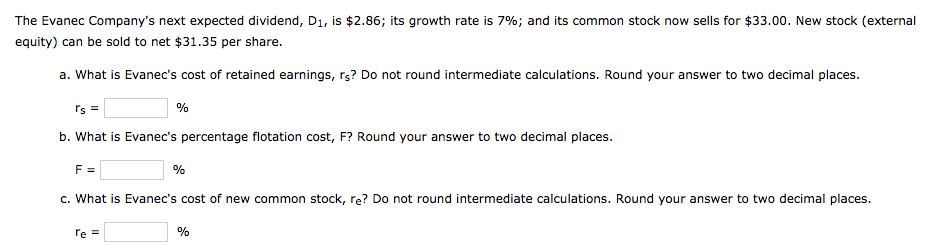The Evanec Company's next expected dividend, D1, is \$2.86; its growth rate is 7%; and its common stock now sells for \$33.00. New stock (external equity) can be sold to net \$31.35 per share. a. What is Evanec's cost of retained earnings, rs? Do not round intermediate calculations. Round your answer to two decimal places. Is = b. What is Evanec's percentage flotation cost, F? Round your answer to two decimal places. F= % c. What is Evanec's cost of...

• ### eBook The Evanec Company's next expected dividend, D1, is \$2.74; its growth rate is 7%; and...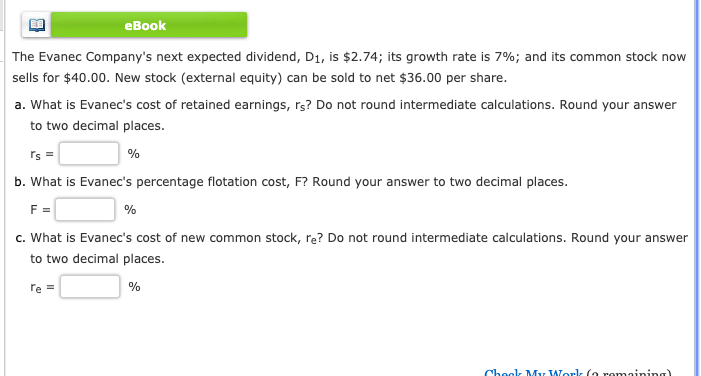eBook The Evanec Company's next expected dividend, D1, is \$2.74; its growth rate is 7%; and its common stock now sells for \$40.00. New stock (external equity) can be sold to net \$36.00 per share. a. What is Evanec's cost of retained earnings, rs? Do not round intermediate calculations. Round your answer to two decimal places. rs = % b. What is Evanec's percentage flotation cost, F? Round your answer to two decimal places. F % c. What is Evanec's...